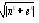#### 若有代数式(其中e仅代表自然对数的底数，不是变量)，则以下能够正确表示该代数式的C语言表达式是(　　)。

• A.sqrt(fabs(pow(n，x)＋exp(x)))
• B.sqrt(fabs(pow(n，x)＋pow(x，e)))
• C.sqrt(abs(n^x＋e^x))
• D.sqrt(fabs(pow(x，n)＋exp(x)))2023年计算机等级考试题库